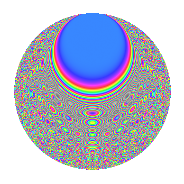# Properties

 Label 525.4.zLevel $525$ Weight $4$ Character orbit 525.z Rep. character $\chi_{525}(64,\cdot)$ Character field $\Q(\zeta_{10})$ Dimension $352$ Sturm bound $320$

# Related objects

## Defining parameters

 Level: $$N$$ $$=$$ $$525 = 3 \cdot 5^{2} \cdot 7$$ Weight: $$k$$ $$=$$ $$4$$ Character orbit: $$[\chi]$$ $$=$$ 525.z (of order $$10$$ and degree $$4$$) Character conductor: $$\operatorname{cond}(\chi)$$ $$=$$ $$25$$ Character field: $$\Q(\zeta_{10})$$ Sturm bound: $$320$$

## Dimensions

The following table gives the dimensions of various subspaces of $$M_{4}(525, [\chi])$$.

Total New Old
Modular forms 976 352 624
Cusp forms 944 352 592
Eisenstein series 32 0 32

## Trace form

 $$352q + 336q^{4} + 28q^{5} + 792q^{9} + O(q^{10})$$ $$352q + 336q^{4} + 28q^{5} + 792q^{9} - 8q^{10} + 24q^{15} - 1000q^{16} - 72q^{19} + 900q^{20} + 84q^{21} - 940q^{22} + 440q^{23} + 488q^{25} - 696q^{26} + 1160q^{29} + 312q^{30} - 960q^{33} - 336q^{34} - 56q^{35} - 3024q^{36} - 2060q^{37} - 624q^{39} - 4836q^{40} - 1192q^{41} + 1596q^{44} - 252q^{45} + 1384q^{46} + 3440q^{47} - 17248q^{49} + 1148q^{50} - 3264q^{51} + 6020q^{53} - 2648q^{55} - 6640q^{58} - 816q^{59} + 228q^{60} + 328q^{61} - 10700q^{62} + 1176q^{64} - 7748q^{65} - 2544q^{66} + 720q^{67} + 1248q^{69} + 756q^{70} + 2128q^{71} + 2880q^{73} - 2336q^{74} - 528q^{75} - 6728q^{76} - 1120q^{77} - 696q^{79} + 332q^{80} - 7128q^{81} - 4920q^{83} - 1008q^{84} - 2908q^{85} - 7320q^{86} - 4560q^{87} - 10620q^{88} + 2484q^{89} + 72q^{90} + 1456q^{91} + 8600q^{92} + 10852q^{94} + 3560q^{95} - 1740q^{96} - 5280q^{97} + O(q^{100})$$

## Decomposition of $$S_{4}^{\mathrm{new}}(525, [\chi])$$ into newform subspaces

The newforms in this space have not yet been added to the LMFDB.

## Decomposition of $$S_{4}^{\mathrm{old}}(525, [\chi])$$ into lower level spaces

$$S_{4}^{\mathrm{old}}(525, [\chi]) \cong$$ $$S_{4}^{\mathrm{new}}(25, [\chi])$$$$^{\oplus 4}$$$$\oplus$$$$S_{4}^{\mathrm{new}}(75, [\chi])$$$$^{\oplus 2}$$$$\oplus$$$$S_{4}^{\mathrm{new}}(175, [\chi])$$$$^{\oplus 2}$$

## Hecke characteristic polynomials

There are no characteristic polynomials of Hecke operators in the database